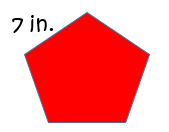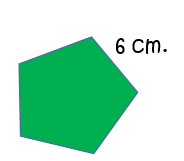# How to Find the Perimeter of a Regular Pentagon

Instructions:

question 1 of 3

### Find the perimeter of this regular pentagon.Create Your Account To Take This Quiz

As a member, you'll also get unlimited access to over 79,000 lessons in math, English, science, history, and more. Plus, get practice tests, quizzes, and personalized coaching to help you succeed.

Try it risk-free for 30 days. Cancel anytime

### 1. Find the perimeter of this regular pentagon.### 2. Find the perimeter for a regular pentagon where each side measures 11 centimeters.

Create your account to access this entire worksheet
Quizzes, practice exams & worksheets
Certificate of Completion
Create an account to get started

Questions about finding a perimeter are not uncommon in both geometry and real life. Complete this short assessment to test your skills at finding the perimeter for regular pentagons.

## Quiz & Worksheet Goals

With this assessment, you will test your abilities in the following:

• Using addition and multiplication to find the perimeter of regular pentagons
• Determining what's needed to find these perimeters
• Finding the values of these perimeters

## Skills Practiced

The skills this assessment helps you practice include:

• Interpreting information - verify that you can read information regarding the perimeters of regular pentagons and interpret it correctly
• Problem solving - use acquired knowledge to solve practice problems that will ask you to find the perimeter of regular pentagons
• Making connections - use your understanding of the concept of perimeter to help yourself solve problems

The lesson that comes with this assessment, How to Find the Perimeter of a Regular Pentagon, will show you more about this math task, as well as information on the following:

• Define what a regular pentagon is
• Demonstrate the difference between regular and irregular pentagons
• Give real-life examples of how finding perimeters can be used
Final ExamMath for Kids
Status: Not Started
Chapter ExamGeometry for Elementary School
Status: Not Started

Support